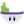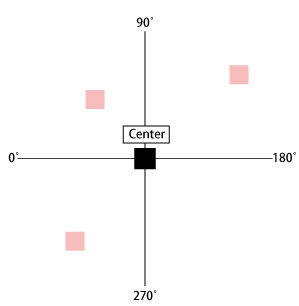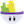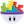#### Howdy, Stranger!

It looks like you're new here. If you want to get involved, click one of these buttons!

# Have any function can count angle(direction)?

Posts: 215Member, PROedited April 2019

Hello everyone.
I have a math problem.
Have any function can count red actor's angle(direction)?
Thank you.

For example:
The center object's position.x = 50 and position.y = 50.
The red object's position.x = 25 and position.y = 20
As for center object, have any function can count red actor's angle(direction)?• Posts: 194Member, PROYou need an exact angle number or just the direction like left, right, up, down?

If only 4 directions then it’s easy by checking the actor’s velocity. If x > 0 then it’s right, and smaller is left. If y > 0 the it’s up and smaller is down.

If you need the exact angel number then I’ll need to leave it for a higher skill developerMy Gamesalad Games On App Store:

• Posts: 409Member, PRO@Weiyu said:
Hello everyone.
I have a math problem.
Have any function can count red actor's angle(direction)?
Thank you.

For example:
The center object's position.x = 50 and position.y = 50.
The red object's position.x = 25 and position.y = 20
As for center object, have any function can count red actor's angle(direction)?use asin/acos/atan, using the x and y displacement between each point as the lengths of a pythagoras/right angle triangle. you would also need a real attribute to allocate the value to when it is calculated.

sin (angle) = length of opposite side to angle/length of hypotenuse
cos (angle) = length of adjacent side to angle/length of hypotenuse
tan (angle) = length of opposite side to angle/length of adjacent side to angle

the a function mentioned above calculates the angle that is the object of whatever of sin/cos/tan you are using.

to get the length of adjacent or opposite sides, you use the y position of one, minus the y position of the other (or x position depending if the side you are using runs along the x or y axis). if using the hypotenuse, you would need to use the magnitude function between the two points. easiest way though i find is atan (so your variable function in your scene, call it (maybe) angle would be

change attribute angle to atan ((y.positionOfActor1-y.positionOfActor2)/(x.positionOfActor1-x.positionOfActor2))

i think that's what you're asking so i hope that makes sense.

• Posts: 409Member, PROpractical demo file attached for reference

• Posts: 194Member, PRO@bob loblaw this is what I meant "a higher skill developer". How the hell in the earth you figured out that complicated equation?My Gamesalad Games On App Store:

• Posts: 409Member, PRO@AlkaPP said:
@bob loblaw this is what I meant "a higher skill developer". How the hell in the earth you figured out that complicated equation?hahaa cheers. it's from high school (year 10) maths a long time ago. my teacher had a thing to remember it, and i can't believe it's stuck with me for this long.

some old hags can always hide their old age

sin=o/h cos=a/h tan=o/a

hope it can help a few other people as well. very useful function for rotating projectiles to the right angle along their flight path, as well as an AI function if you have an enemy shooting at a position a player was in at the time of shooting. i'm currently finalising a game inspired by crush the castle/angry birds that uses it in some parts.

props to you mrs. h, by the off chance you ever stumble upon and read this.

• Posts: 575Member, BASICDoes the vector to angle function not do what you want?

Best,
Two.E

• Posts: 409Member, PROhave never used it. will have to give it a go.

• Posts: 575Member, BASICVectorToAngle(center.X - actor.x.pos, center. Y - actor.y.pos)

And if you want the opposite direction, ie the angle facing the middle, then add +180 at the end of the expression.

I assume the function does the maths @bob loblaw described....but I don't know for sure.

Best,
Two.E

• Posts: 409Member, PRO@Two.E said:
VectorToAngle(center.X - actor.x.pos, center. Y - actor.y.pos)

And if you want the opposite direction, ie the angle facing the middle, then add +180 at the end of the expression.

I assume the function does the maths @bob loblaw described....but I don't know for sure.

Best,
Two.E

i think you’re right. looking at that it looks like it’s pretty much the same thing, but with its own easy to understand name.

• Posts: 2,836Member, Sous Chef, PROI believe "VectorToAngle()" is GameSalad's version of "atan2". The great thing about it is that "VectorToAngle()" gives the result in degrees instead of radians.

• Posts: 2,836Member, Sous Chef, PROMaybe this 'blast from the past' might be useful: